085-1263404

### My Journal

All things Mathematical
18 Sep 2018
##### Question 1

A particle moves along a straight line and its distance in feet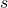from a fixed point in the line is given by the formula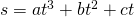where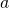,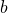,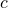are constants anddenotes the time in seconds. The distance is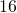when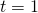and the velocity is zero when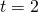and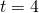. Find the values of,,and verify that the acceleration is zero when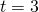. Draw rough diagrams showing how (a) the acceleration, (b) the velocity varies between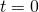and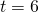.

##### Question 2

A body weighinglb. is moving in a straight line with Simple Harmonic Motion. If the greatest velocity is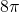ft. per second. and the amplitude of the oscillation isft., find the period of oscillation and the force of attraction towards the centre when the body is at its greatest distance from the centre.

##### Question 3

A body is projected with velocity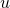, at an inclination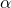to the horizon, from the foot of a plane inclined at angle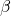to the horizon. Determine its range up the plane. Show that for a given value of, the range is a maximum when the direction of projection bisects the angle between the inclined plane and the vertical. Determine the least velocity with a ball can be thrown to reach the top of a cliff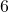ft. high and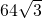ft. away from the thrower, neglecting air resistance.

##### Question 4

If the velocities and accelerations of a number of particles be know, how many the velocity and acceleration of their centre of gravity be found?

A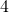lb. weight is placed on a smooth table and is attached to a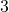lb. weight, which vertically by a light inextensible string passing over a smooth pulley at the edge of the table. Show that the acceleration of the centre of gravity of the two weights is constant in magnitude and direction, and that the centre of gravity moves in a straight line.

##### Question 5

A car weighing one tone is rounding a curve of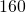yards radius on a level road. What is the greatest speed at which this is possible, without causing the car to overturn, if the wheel gauge is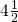ft. and the C.G. is mid-way between the wheels transversely and at a height offt. from the ground? What is the frictional force between the road and tyres at this speed?

##### Question 6

An engine pumps one ton of water per minute to a height of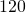ft. and delivers it through a pipe whose cross-sectional area issquare inches. Find (i) the work done against gravity per minute in ft. lbs.; (ii) the kinetic energy of the water delivered in one minute in ft. lbs.; (iii) the Horse Power of the engine, neglecting all losses due to friction. (A cubic foot of water weights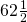lbs.)

##### Question 7

A pin-jointed framework of the form shown in diagram, is pin-jointed to a vertical wall at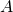and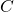and carries a vertical load ofton at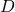. Find the forces exerted by the frame on the wall, and determine the stresses in the bards, indicating whether the bar is in thrust or in tension in each case.##### Question 8

A weight of half-a-tone is allowed to fall freely through a height of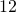ft. to drive a pile weighing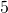cwt. into the ground. Find the average resistance to the motion of the pile if it is driven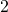inches by the blow, assuming that the weight moves on with the pile.

##### Question 9

A body, weighinglbs., is kept in equilibrium on a rough plane inclined at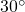to the horizontal by a cord inclined atto the plane. Find the frictional force between the body and the plane and indicate its direction when the tension in the cord is 6lbs. Find the work done in dragging the body slowly through a distance of one foot up the plane, assuming the coefficient of friction to beand the cord to remain constantly at an angle ofto the plane.

##### Question 10

The two balls,and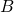, of a governor, weigh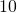lbs. each; the arms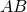and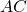are each inclined atto the vertical and areft. long. Find the number of revolutions per minute they are making and the tension in each rod; the weights of the rods,,[sic], may be neglected.

##### Citation:

State Examinations Commission (2018). State Examination Commission. Accessed at: https://www.examinations.ie/?l=en&mc=au&sc=ru

Malone, D and Murray, H. (2016). Archive of Maths State Exams Papers. Accessed at: http://archive.maths.nuim.ie/staff/dmalone/StateExamPapers/

##### Licence:

“Contains Irish Public Sector Information licensed under a Creative Commons Attribution 4.0 International (CC BY 4.0) licence”.

The EU Directive 2003/98/EC on the re-use of public sector information, its amendment EU Directive 2013/37/EC, its transposed Irish Statutory Instruments S.I. No. 279/2005, S.I No. 103/2008, and S.I. No. 525/2015, and related Circulars issued by the Department of Finance (Circular 32/05), and Department of Public Expenditure and Reform (Circular 16/15 and Circular 12/16).

Note. Circular 12/2016: Licence for Re-Use of Public Sector Information adopts CC-BY as the standard PSI licence, and notes that the open standard licence identified in this Circular supersedes PSI General Licence No: 2005/08/01.

Links:

https://circulars.gov.ie/pdf/circular/per/2016/12.pdf

https://creativecommons.org/licenses/by/4.0/legalcode

This site uses Akismet to reduce spam. Learn how your comment data is processed.